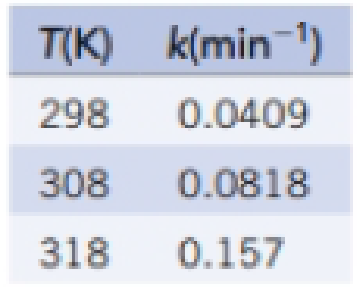Chapter 14, Problem 73GQ

Chapter
Section
Textbook Problem

Data for the reaction[Mn(CO)5(CH3CN)]+ + NC5H5 → (Mn(CO)5(NC5H5)]+ + CH3CNare given in the table. Calculate Ea from a plot of In k versus 1/T.Interpretation Introduction

Interpretation:

The value for Ea should be determined using the plot obtained by the given set of data.

Concept introduction:

Rate law: It is generally the rate equation that consists of the reaction rate with the concentration or the pressures of the reactants and constant parameters.

Rate constant: The rate constant for a chemical reaction is the proportionality term in the chemical reaction rate law which gives the relationship between the rate and the concentration of the reactant present in the chemical reaction.

Rate order: The order of each reactant in a reaction is represented by the exponential term of the respective reactant present in the rate law and the overall order of the reaction is the sum of all the exponents of all reactants present in the chemical reaction.  The order of the reaction is directly proportional to the concentration of the reactants.

Arrhenius equation:

• Arrhenius equation is a formula that represents the temperature dependence of reaction rates
• The Arrhenius equation has to be represented as follows

k=AeEa/RTlnk=lnAeEa/RTlnk=(EaR)(1T)+lnA

• Ea represents the activation energy and it’s unit is kJ/mol
• R represents the universal gas constant and it has the value of 8.314 J/K.mol
• T represents the absolute temperature
• A represents the frequency factor or collision frequency
• e represents the base of natural logarithm
•  Arrhenius equation equation was proposed by Svante Arrhenius in 1889.
Explanation

For the given reaction lnk and 1/T are tabulated as follows by using the given set of data.

The corresponding graph is as follows,

Findthe slope for any two values present in the graph:slope=-1.852-(-2.503)0.003145-0

Still sussing out bartleby?

Check out a sample textbook solution.

See a sample solution

The Solution to Your Study Problems

Bartleby provides explanations to thousands of textbook problems written by our experts, many with advanced degrees!

Get Started

Find more solutions based on key concepts